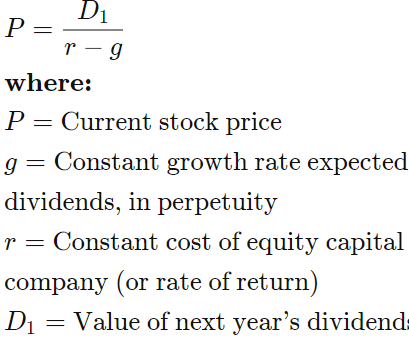# Hart entreprises recently paid a dividend, D0, of 1.25$. the company expected to grow at a... ## Question: Hart entreprises recently paid a dividend, D0, of 1.25$. the company expected to grow at a constant rate of 5%. the firm's cost of equity is 10%.

How far away is the horizon date?

What is the firm's horizon or continuing, value?

What is the firm's intrinsic value today?

## Dividend Discount Model:

This problem calls for a basic understanding of the constant growth dividend discount model, which is also known as the Gordon growth model (GGM). It is a popular method for valuing equity investments. The GGM assumes the fair value of a stock is the sum of all future dividends, discounted to the present day using the relevant cost of equity.

To answer the three questions posed, we first must clarify some terminology, namely horizon date, horizon value, and intrinsic value. Brief definitions are outlined below.

- Horizon date is the date a company's growth rate becomes constant.

- Horizon value is the value of a company at the point its growth rate becomes constant.

- Intrinsic value is the inherent value of an investment. According to the constant growth dividend discount model, this is the sum of all future dividends, discounted to the present day using the relevant cost of equity.

For this problem, we are told Hart is expected to grow at a constant rate of 5%. So, this means (1) we are already at the horizon date and (2) the horizon value and today's intrinsic value are one in the same. Let's solve for this value using the dividend discount model (DDM), which is illustrated below.We are given the following information:

D0 = $1.25 g = 5.0% r = 10.0% To solve for P0, we first must determine D1, the dividend expected in one year. It's computed as follows: D1 = D0(1+g) D1 =$1.25(1.05) = $1.31 Now, we can plug the known information into the DDM to solve for P0. The computation is below. P0 =$1.31 / (.10-.05)

P0 = \$26.20